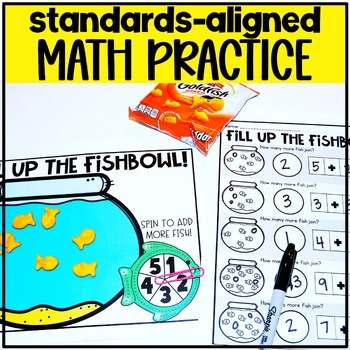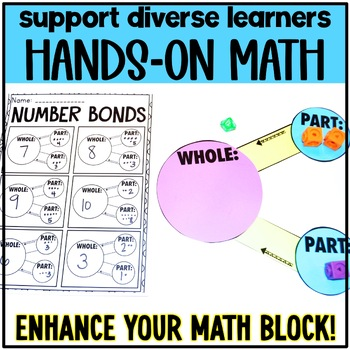Rated 4.94 out of 5, based on 736 reviews
736 Ratings;
1st
Subjects
Resource Type
Standards
Formats Included
• Zip
Pages
250+
\$15.00
\$15.00
Report this resource to TPT

#### What educators are saying

Our school's math program is a little "dry" so this program gives my math pizazz!!! I love it. Thank you for putting learning and fun hand in hand!
I LOVE this unit! It is a life saver planning wise and so incredibly engaging for the kiddos! Best money I've spent on a math resource!
##### Also included in
1. First Grade teachers are you looking to spruce up your math block? Are you tired of digging for materials, lessons, and activities that are just mediocre? Magic of Math for First Grade is a series of math units that can be used as a stand alone curriculum OR the activities can be used to supplem
Price \$123.30Original Price \$136.50Save \$13.20

### Description

Are you ready to introduce addition to your first graders with engaging lesson plans and hands-on addition activities, games, and printables? You're in luck! This complete addition unit helps teach addition strategies using anchor charts, posters, word problems, fact practice, and much more!

The Magic Of Math Unit 2 for FIRST GRADE focuses on:

Week 4: Addition Word Problems and Fact Practice

*Please Note: This is an INTRODUCTION to Addition. We will be focusing on Addition again in Unit 5. We will dig much deeper into addition in Unit 10.

For Other 1st Grade Units Click Below:

1st Grade Magic of Math Unit One: Place Value

1st Grade Magic of Math Unit Three: Subtraction

1st Grade Magic of Math Unit Four: Shapes and Fractions

1st Grade Magic of Math Unit Five: Mid-Year Mix Up

1st Grade Magic of Math Unit Six: Nonstandard Measurement

1st Grade Magic of Math Unit Seven: Telling Time

1st Grade Magic of Math Unit Eight: Graphing

1st Grade Magic of Math Unit Nine: Money

1st Grade Magic of Math Unit Eleven: End of the Year Review

Here's what to expect from this month long unit for 1st grade:

-Daily Lesson Plans for 20 days

-Aligned with the 1st Grade Common Core and TEKS

-20 Word Problems for each day that complement the skills

-Interactive Notebook Printables and Independent Works

-Games, Activities, and Crafts to enhance learning

-Minilessons with ideas on how to teach the skills as well as materials needed

-Vocabulary Cards

-I Can Statements

-Assessments

-Anchor Chart and Pocket Chart Materials

Want a Closer Look? Here are some (not all!) activities included:

- I Can Add Numbers Students Work Mat

- Grab and Go Addition Game

- Part Part Whole Number Bond Chart

- Number Bond Cards

- Number Bond Work Mat for Students

- Number Bond Printables

- Number Bond Matching

- Spin to Join

- I Can Add to a Set Work Mat

- Fill Up the Fishbowl Game

- The Dino Stomp Minilesson

- Dino Feet Word Problem

- Dino Domino Dash Interactive Notebook

- True or False Group Activity

- Addition Bracelets and Find a Friend

- Adding Zero Interactive Notebook or Printable

- Counting On With Cubes Lesson

- Counting On Number Line Scroll

- I Can Count On Activity

- Giant Number Line Counting On Activity

- Musical Math: Counting On

- Number Line Interactive Notebooks

- Giant Making Tens Ten Frame Activity and lesson

- Spin for 10 activity (finding the missing addend)

- Ways to Make 10 with missing addends activity

- Doubles Group Sort

- Double! Double! Monster Teeth game

- Interactive Notebooks: Doubles

- Mystery Numbers (whole group game)

- Monster Mash: missing numbers BINGO

- Printable: Missing Doubles

- Doubles Plus One Charts

- Double Trouble Game (doubles plus one)

- Interactive Notebooks: Doubles Plus One

- Flip Flop Activity

- Missing Numbers in Equations Activity

- PSA bulletin board materials (problem-solving strategy)

- PSA folder templates

- PSA slider

- Plus Pal Hat

- Problem-Solving: Understanding what the problem is asking

- Over 25 word problems for students to solve

- Learning how to read and solve word problems

- Word Problem Solving Mat

- Ninja Kicks Addition Game (differentiated)

- Piece It Together: Word Problem Activity

- Swim to the Top (addition game)

- Problem-Solving: Learning how to check answers

- The Checker: checking problem ideas

- Sunny Sums (differentiated addition game)

- Word Problem Assessment

- Addition Tic Tac Toe (differentiated)

What is the Magic of Math?

The Magic of Math is a series of math lesson plans and activities that can be used as your math curriculum or as a supplement to the program that you are already using. We provide daily lesson plans, word problems, mini-lesson, activities, and interactive notebook entries for four weeks at a time. If you don't have time for it all, that's okay too! You can just pick and choose the pieces that you want to incorporate into your math block!

Are these activities repeated in other units you have?

Absolutely NOT! We create all new activities for each Magic of Math unit. They are all unique to this purchase and are not copied/pasted from things we have previously made.

What is the difference between Magic of Math and other units you have?

Magic of Math is organized as daily lesson plans with everything you need rather than just activities.

WHAT IT IS NOT:

It is NOT a collection of worksheets. Instead, we use games, hands-on activities, engaging mini-lessons, and interactive notebooks to build our curriculum. We do try to keep in mind the amount of prep that is involved with the lessons, but some things will need to be printed, cut, and put together to use in your classroom.

Want to know more about Magic of Math? You can find a blog post about it HERE!

Total Pages
250+
Not Included
Teaching Duration
1 month
Report this resource to TPT
Reported resources will be reviewed by our team. Report this resource to let us know if this resource violates TPT’s content guidelines.

### Standards

to see state-specific standards (only available in the US).
Use addition and subtraction within 20 to solve word problems involving situations of adding to, taking from, putting together, taking apart, and comparing, with unknowns in all positions, e.g., by using objects, drawings, and equations with a symbol for the unknown number to represent the problem.
Apply properties of operations as strategies to add and subtract. If 8 + 3 = 11 is known, then 3 + 8 = 11 is also known. (Commutative property of addition.) To add 2 + 6 + 4, the second two numbers can be added to make a ten, so 2 + 6 + 4 = 2 + 10 = 12. (Associative property of addition.)
Add and subtract within 20, demonstrating fluency for addition and subtraction within 10. Use strategies such as counting on; making ten (e.g., 8 + 6 = 8 + 2 + 4 = 10 + 4 = 14); decomposing a number leading to a ten (e.g., 13 - 4 = 13 - 3 - 1 = 10 - 1 = 9); using the relationship between addition and subtraction (e.g., knowing that 8 + 4 = 12, one knows 12 - 8 = 4); and creating equivalent but easier or known sums (e.g., adding 6 + 7 by creating the known equivalent 6 + 6 + 1 = 12 + 1 = 13).
Understand the meaning of the equal sign, and determine if equations involving addition and subtraction are true or false. For example, which of the following equations are true and which are false? 6 = 6, 7 = 8 - 1, 5 + 2 = 2 + 5, 4 + 1 = 5 + 2.
Determine the unknown whole number in an addition or subtraction equation relating three whole numbers. For example, determine the unknown number that makes the equation true in each of the equations 8 + ? = 11, 5 = ▯ - 3, 6 + 6 = ▯.